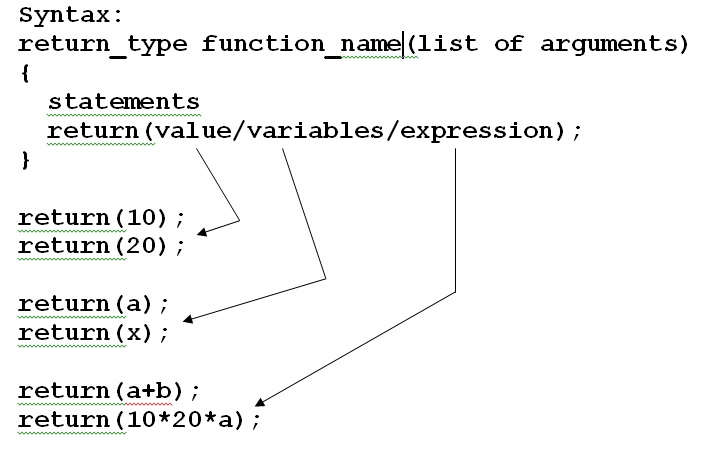# C++ Programming | Functions 2

Function declaration

Function declaration is an indication to the system that the specified function is used later in the program. While giving function declaration we have to mention the following

1.Return type of the function i.e. the type of value of the function is returning back.
2.Name of the function.
3.The list of arguments being passed to the function i.e. list of data types and variable names(optional).
4.The function declaration is terminated by semicolon(;).

Syntax:
Returntype function_name (list of arguments);

Function calling

Function calling means invoking the function i.e. executing the function.
While calling the function we have to specify the following:
1.variable to receive the value, if function is returning some value (optional).
2.Name of the function being called.
3.List of values/variables being passed to the function , if passed.
4.The function calling is terminated by the semicolon.

Syntax:
Variable=function_name(list of values/variables);

Function definition

• The function definition is a set of statements written to perform a specific task.
• The function definition helps us to specify what and how the operation is performed.
• The definition of the function is not terminated by semicolon.
• The function definition can be given either above or below the calling function (main function).
• If the definition of the function is given above the calling function [main ()] then giving function declaration becomes optional.

Syntax: